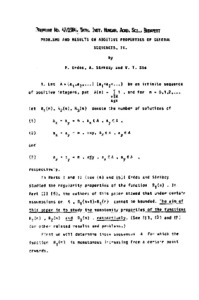REAL

# Problems and Results on Additive Properties of General Sequences IV

Erdős, P. and Sárközy, A. and T. Sós, V. (1985) Problems and Results on Additive Properties of General Sequences IV. In: Number theory : Proceedings of the 4th Matscience Conference held at Ootacamund, India, January 5–10, 1984. Lecture Notes in Mathematics (1122). Springer, Berlin; Heidelberg, pp. 85-104. ISBN 978-3-540-15222-4 (print); 978-3-540-39642-0Preview
Text
1985-25.pdf

Download (1MB) | Preview

## Abstract

Let A={a1,a2,⋯}, a1<a2<⋯, be an infinite sequence of positive integers. One defines its counting function as A(n)=Card{a∈A;a≤n} (n=0,1,2,⋯), and the representation functions R1(n), R2(n), R3(n) (n=0,1,2,⋯), as being the number of representations of n in the form: (1) n=a+a′, a∈A, a′∈A, (2) n=a+a′, a<a′, a∈A, a′∈A, (3) n=a+a′, a≤a′, a∈A, a′∈A, respectively. Of course, for any n≥0, R1(n)=R2(n)+R3(n), and R3(n) is equal either to R2(n)+1, if n is even and n/2 belongs to A, or to R2(n), otherwise. In the first three parts of this series of papers [Erdős and Sárközy, Part I, Pacific J. Math. 118 (1985), no. 2, 347–357; MR0789175 (86j:11015); Part II, Acta Math. Hungar. 48 (1986), 201–211; MR0858398 (88c:11016); Part III, the authors, Studia Sci. Math. Hungar. 22 (1987), no. 1, 53–63], regularity properties of the asymptotic behavior of the function R1 were studied. In Parts IV and V the authors study monotonicity properties of the three functions R1,R2,R3. In Part IV, they prove first that the function R1 is monotone increasing from a certain point on (i.e., there exists an n0 withR1(n+1)≥R1(n) for n≥n0) if and only if the sequence A contains all the integers from a certain point on. The proof uses elementary but complex considerations on counting functions. Secondly, they show that R2 has a different behavior, by exhibiting a class of sequences A satisfying A(n <n−cn1/3 for all large n and such that R2 is monotone increasing from some point onwards. The third result proved in Part IV is that if A(n)=o(n/logn) then the functions R2 and R3 cannot be monotone increasing from a certain point on. Here, the proof is based on analytic properties of the generating function f(z)=∑a∈Aza (|z|<1), corresponding to the sequence A. Part V treats the monotonicity of R3. The main result is as follows: If (4) limn→+∞(n−A(n))/logn=+∞, then lim supN→+∞∑k=1N(R3(2k)−R3(2k+1))=+∞. (Thus, roughly speaking, ai+aj assumes more even values than odd ones.) This theorem implies, firstly, that under hypothesis (4), which is weaker than A(n)=o(n/logn), R3 cannot be monotone increasing from a certain point on, and, secondly, that if A is an infinite "Sidon sequence'' (also called a "B2-sequence'', i.e., a sequence such that R3(n)≤1 for all n), then there are infinitely many integers k such that 2k can be represented in the form 2k=a+a′, a∈A, a′∈A, but 2k+1=a+a′, a∈A, a′∈A, is impossible. Part V finishes with the construction of a sequence showing that the main result is almost best possible. The proofs in Part V are of the same nature as those in Part IV.

Item Type: Book Section Q Science / természettudomány > QA Mathematics / matematika MTMT SWORD MTMT SWORD 26 Jun 2020 12:39 26 Jun 2020 12:39 http://real.mtak.hu/id/eprint/110568

### Actions (login required)Edit Item